# 18年六月计算几何读书笔记

## Bookmark

CGAA: Computational Geometry Algorithms and Applications 3rd

## Line Segment Intersection

2018.6.22, CGAA

1. Entry事件：遇到某条线段$s_j$的entry point时，设此时和sweep line相交的线段按排序为$\dots, s_i, s_j, s_k, \dots$，则$s_j$变得和$s_i$以及$s_k$相邻。

2. Exit事件：遇到某条线段$s_j$的exit point时，若原来和sweep line相交的线段排序为$\dots, s_i, s_j, s_k, \dots$，则$s_i$和$s_k$变为相邻。

3. 交点事件：遇到两条线段$s_i$和$s_j$的交点时，若原来和sweep line相交的线段排序为$\dots, s_h, s_i, s_j, s_k, \dots$，则$s_h$和$s_j$变为相邻，$s_i$和$s_k$变为相邻。

## Doubly-Connected Edge List

2018.6.22, CGAA

1. 遍历某个面的所有边
2. 遍历通过某个顶点的所有边
3. 通过一个面的某条边访问占据该边的另一个面

DCEL大概长这样：

struct vertex_t;
struct fce_t;

struct half_edge_t
{
half_edge_t *succ, *prev, *twin;
vertex_t *origin;
face_t *face;
};

struct vertex_t
{
point2f coord;
half_edge_t *edge;     // arbitrary edge that has the vertex as its origin
};

struct face_t
{
half_edge_t *edge;     // arbitrary edge of its outer boundary
list<edge*> int_edges; // one for each hole in the interior
};


2018.6.22, CGAA

Simple Polygon: regions enclosed by a single closed polygonal chain that does not intersect itself.

Diagonal: an open segment that connects two vertices and lies in the interior of the polygon.

Triangulation (of polygon): a decomposition into triangles by a maximal set of non-intersecting diagonals.

Theorem. Every simple polygon admits a triangulation, and any triangulation of a simple polygon with $n$ vertices consists of exactly $n - 2$ triangles.

Art Gallery Theorem. For a simple polygon with $n$ vertices, $\lfloor n / 3 \rfloor$ cameras are occasionally necessary and always sufficient to have every point in the polygon visible from at least one of the cameras.

## Overlay of Two Subdivisions

2018.6.23, CGAA

$\mathcal O(\mathcal S_1, \mathcal S_2)$的计算方法和所使用的subdivision表示方法息息相关，在这里假设是输入输出都用DCEL表示。容易证明两个输入DCEL中没有发生相交的那些half edge、face和vertex都可以原封不动地拷贝到输出DCEL中，需要重新计算的仅仅是$\mathcal S_1$和$\mathcal S_2$之间发生了相交的部分。

1. 每个boundary对应$\mathcal G$中的一个顶点，此外对subdivision最外面的那个unbounded “face”，也设置一个对应的顶点。
2. 对两个boundaries，若其中一个是boundary of a hole，而另一个boundary中恰有half edge是hole boundary的lowest leftmost vertex左方最近的一条边，则这两个boundary对应的顶点间有一条边。若某个boundary地lowest leftmost vertex左侧没有half edge，它就和unbounded face对应地节点链在一起。

Lemma. $\mathcal G$中的每个连通分量恰对应一个face的boundary集合。

## Polygon Triangulation

2018.6.23, CGAA

### Polygon into Monotone Pieces

Monotone: a simple polygon is called monotone with respect to a line $\ell$ if for any line $\ell’$ perpecdicular to $\ell$ the intersection of the polygon with $\ell’$ is connected. A polygon that is monotone with respect to the $y$-axis is called $y$-monotone.

Turn Vertex: 相邻的两条边都朝下或者都朝上的顶点称为turn vertex。把多边形转为monotone的过程也正是去除turn vertex的过程。

Below & Above: a point $p$ is below another point $q$ iff $(p_y < q_y)$ or $(p_y = q_y \wedge p_x > q_x)$. A point $p$ is above another point $q$ iff $(p_y > q_y)$ or $(p_y = q_y \wedge p_x < q_x)$。也就是说先按$y$坐标从下到上排序，对$y$相同的点再按$x$坐标从右往左排序，“左上”的点是最“高”的。

Start Vertex: a vertex $v$ is a start vertex iff its neighbors lie below it and the interior angle at $v$ is less that $\pi$.

Split Vertex: a vertex $v$ is a split vertex iff its neighbors lie below it and the interior angle at $v$ is greater than $\pi$.

End Vertex: a vertex $v$ is an end vertex iff its neighbors lie above it and the interior angle at $v$ is less than $\pi$.

Merge Vertex: a vertex $v$ is a merge vertex iff its neighbors lie above it and the interior angle at $v$ is greater than $\pi$.

Lemma. a polygon is $y$-monotone if it has no split vertices or merge vertices.

Helper: 对某条边$e_j$，定义$\mathrm{helper}(e_j)$为

$\arg \min_{v_y}\{v\mid \ell_y < v_y \le \mathrm{up} (e_j), \mathrm{HorSeg}(v, e_j) \text{ in } \mathcal P\}$

Merge vertex的处理要更困难一些，因为它连接的对象是在sweep line的下方，即相关顶点的选择必须被推迟。设$e_j$是merge vertex $v_i$左侧最近边，则当sweep line扫描到$v_i$时，$v_i$必然成为$e_j$的helper顶点。在之后的扫描中，如果再次更新了$e_j$的helper，那么把$v_i$和$e_j$的新helper连接起来即可；否则，可以把$v_i$和$e_j$的下端点连接起来。所以，只需要每次更新某条边的helper或遇到某条边的下端点时，检查该边原本的helper是否时一个merge vertex即可。

proedure make_polygon_into_monotone(P)
for each vertex v[i] in P, ordered by desc y coord
handle(v[i])

procedure handle_start_vertex(v[i])
helper(e[i]) = v[i]

procedure handle_end_vertex(v[i])
if(helper(e[i-1]) is a merge vertex)
connect(helper(e[i-1]), v[i])

procedure handle_split_vertex(v[i])
let e[j] = DirectLeft(v[i])
connect(helper(e[j]), v[i])
helper(e[j]) = v[i]
helper(e[i]) = v[i]

procedure handle_merge_vertex(v[i])
if(helper(e[i-1]) is a merge vertex)
connect(helper(e[i-1]), v[i])
let e[j] = DirectLeft(v[i])
if(helper(e[j]) is a merge vertex)
connect(helper(e[j]), v[i])
helper(e[j]) = v[i]

procedure handle_regular_vertex(v[i])
if the interior of P lies to the right of v[i]
if(helper(e[i-1]) is a merge vertex)
connect(helper(e[i-1]), v[i])
helper(e[i]) = v[i]
else
let e[j] = DirectLeft(v[i])
if(helper(e[j]) is a merge vertex)
connect(helper(e[j]), v[i])
helper(e[j]) = v[i]


Theorem. a simple polygon with $n$ vertices can be partitioned into $y$-monotone polygons in $\mathrm O(n\log n)$ time with an algorithm that uses $\mathrm O(n)$ storage.

### Triangulating a Monotone Polygon

把P的顶点分为左链和右链，并把所有顶点从上到下排序，记排序结果为u[1..n]
procedure triangulate_monotone_polygon(u)
stack.clear()
for j = 3 to n-1
if u[j].chain != stack.top().chain
while stack.size() > 1
connect(u[j], stack.pop())
stack.push(u[j-1])
stack.push(u[j])
else
last_popped = stack.pop()
while !stack.empty()
last_popped = stack.pop()
if connect(last_popped, u[j]) != successful
break;
stack.push(last_popped)
stack.push(u[j])
add diagonals from u[n] to all stack vertices except the first and the last


Theorem. a simple polygon with $n$ vertices can be triangulated in $\mathrm O(n\log n)$ time.

## Point Location Query

2018.6.24, CGAA

### Trapezoidal Map

Non-crossing: 称两条平面线段是不相交的，当且仅当它们的交集为空或只包含自己的端点。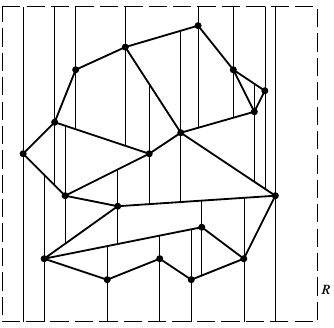Lemma. Each face in a trapezoidal map of a set $\mathcal S$ of line segments in general position has one or two vertical sides and exactly two non-vertical sides.

Top & Bottom: 每个梯形$\Delta$一定有两个non-vertical sides，上面那个所对应的原$\mathcal S \cup R$中的边记作$\mathscr T(\Delta)$，下面那个对应的原$\mathcal S \cup R$中的边记作$\mathscr B(\Delta)$。

Left & Right: 除了整个$\mathcal T(\mathcal S)$中最左侧和最右侧的两个矩形外，每个梯形$\Delta$左侧如果有条垂直边，那么记引出该垂直边的那个原$\mathcal S$中的顶点为$\mathscr L(\Delta)$，否则记$\mathscr T(\Delta)$和$\mathscr B(\Delta)$的唯一交点（位于$\Delta$的左侧）为$\mathscr L(\Delta)$。类似地，可以定义$\mathscr R(\Delta)$。

Lemma. the trapezoidal map $\mathcal T(\mathcal S)$ of a set $\mathcal S$ of $n$ line segments in general position contains $\mathrm O(n)$ vertices and $\mathrm O(n)$ trapezoids.

### Search Structure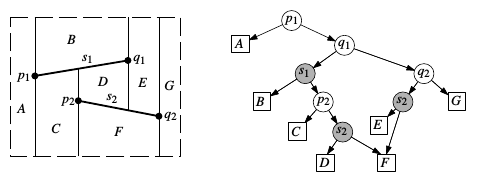procedure trapezoidal_map(S: set of n non-crrssing line segments)
make R
init T as trapezoids of empty set
init D with a single leaf node corresponding to R
compute a random permutation s[1..n] of the segments in S
for i = 1 to n
find T[0..k] of trapezoids in T properly intersected by s[i]
remove T[0..k] in T
insert new trapezoids that appear because of the insertion of s[i] and T[0..k]
remove leaves of T[0..k] in D
create leaves for the new trapezoids
link the new leaves to the existing inner nodes in D


procedure find_Ts(T, D, s[i])
let p = left end point of s[i]
let q = right end point of s[i]
search with p in the search structure D to find T
j = 0
while q lies to the right of R(T[j])
if R(T[j]) lies above s[i]
let T[j+1] = lower right neighbor of T[j]
else
let T[j+1] = upper right neighbor of T[j]
j = j + 1
return T[0..j]


### Degenerate Cases

$\varphi : \left( \begin{matrix} x \\ y \end{matrix} \right) \mapsto \left( \begin{matrix} x + \varepsilon y \\ y \end{matrix} \right)$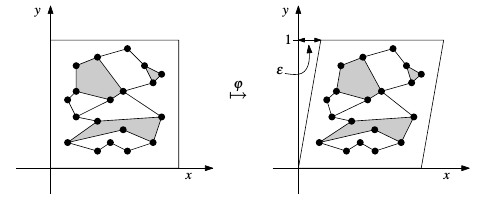Theorem. algorithm trapezoidal_map computes the trapezoidal map $\mathcal T(\mathcal S)$ of a set $\mathcal S$ of $n$ non-crossing line segments and a search structure $\mathcal D$ for $\mathcal T(\mathcal S)$ om $\mathrm O(n\log n)$ time. The expected size of $\mathcal D$ is $\mathrm O(n)$ and for any query point $q$ the expected query time is $\mathrm O(n\log n)$.

## Voronoi Diagram

2018.6.24, CGAA

### Definitions & Properties

Euclidean Distance: 平面上两点$p, q$间的欧氏距离定义为

$\mathrm{dist}(p, q) = \sqrt{(p_x - q_x)^2 + (p_y - q_y)^2}$

Voronoi Diagram: 给定平面点集$P = \{ p_1, \dots, p_n \}$，$P$所定义的Voronoi Diagram（维诺图）是一个planar subdivision，每个face对应一个$p_i$。平面上一点$p$位于某个$p_i$的face内，当且仅当

$\forall p_j \in P, p_j \ne p_i \Rightarrow \mathrm{dist}(p, p_i) < \mathrm{dist}(p, p_j)$

Cell: $\mathcal V(p_i) := \bigcap_{1 \le j \le n, j \ne i}h(p_i, p_j)$，其中$h(p, q)$是以点$p, q$中线为边界的$p$那一边的半平面，即：

$\mathcal V(p_i) := \bigcap_{1 \le j \le n, j \ne i}\{r \mid \mathrm{dist}(r, p_i) < \mathrm{dist}(r, p_j)\}$

Theorem. for $n \ge 3$, the number of vertices in the Voronoi diagram of a set of $n$ point sites in te plane is at most $2n-5$ and the number of edges is at most $3n-6$.

Cp: 定义$C_P(p)$为以$p \in \mathbb R^2$为中心的、内部不包含其他任何顶点的圆。显然$C_P(p)$是个闭集。

Theorem. 点$q \in \mathbb R^2$是$\mathrm{Vor}(P)$的一个顶点，当且仅当$C_P(q)$边界上有不少于3个$P$中的点。

Theorem. $p_i, p_j$连线的垂直平分直线的一个子线段是$\mathrm{Vor}(P)$的一条边，当且仅当存在点$q \in \mathbb R^2$使得$C_P(q) \cap P = \{p_i, p_j\}$。

### Computation

Fortune算法也是基于sweep line的，它的框架和之前的线段求交算法有些相似——也有event point的概念。在sweep line $\ell$移动的过程中，要保证$\mathrm{Vor}(P)$在$\ell$上方的部分都已被计算出来是不现实的，这是由于这部分可能依赖于$\ell$下方的、还未被扫描到的点。Fortune退而求其次，维护任一时刻$\mathrm{Vor}(P)$在$\ell$上方、且和$\ell$下方顶点无关的部分。

$\mathrm{dist}(q, \ell) \ge \min_{p \in P \cap \ell^+}\mathrm{dist}(p, q)$

$\beta_\ell = \{\beta_p = \{q \mid \mathrm{dist}(p, q) = \mathrm{dist}(\ell, q)\} \mid p \in P \cap \ell^+\}$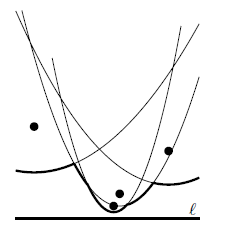Observation. the beach line is $x$-monotone, that is, every vertical line intersects it in exactly one point.

Site事件: $\ell$在扫描过程中遇到一个$p \in P$，称为一个site事件。Site事件会产生两个新断点以及一个arc。事实上，这也是唯一能够产生新arc的情景——

Lemma. 一个新arc只可能在site事件中出现。

Circle事件: 对形成beach line上相邻的三段arc的的三个$P$中的点，称$\ell$和它们所确定的圆相切为一个circle事件。

Lemma. 一个已有的arc只可能在circle事件中消失。

Theorem. the Voronoi diagram of a set of $n$ point sites in the plane can be computed with a sweep line algorithm in $\mathrm O(n\log n)$ time using $\mathrm (n)$ storage.

## Delaunay Triangulations

2018.6.25

Triangulation: a triangulation of $P = \{p_1, \dots, p_n\} \subset \mathbb R^2$ is a maximal planar subdivision whose vertex set is $P$.

Theorem. 设$P$中包含$n$个（不全共线的）顶点，其中有$k$个顶点位于构成$P$的凸包的多边形上，则$P$的任何三角化结果都包含$2n-n-k$个三角形，$3n-3-k$条边

Angle Vector: 任给点集$P$的一个三角化结果$\mathcal T$，若其中共包含$m$个三角形，则$m$个三角形共有$3m$个内角。这些内角非降序排列的结果$A(\mathcal T) := (\alpha_1, \alpha_2, \dots, \alpha_{3m})$称为$\mathcal T$的角度向量。定义同一点集$P$的不同三角化结果的角度向量间的全序关系为角度的词法序。称$P$的三角化结果$\mathcal T$是角度最优的，当且仅当$A(\mathcal T)$不小于任何其他$P$的三角化结果的角度向量。

Thale’s Theorem. 设直线$\ell$与圆$C$交于$a, b$两点，$p, q, r, s$落在$\ell$的同一侧。若$p, q$在$C$上，$r$在$C$内，$s$在$C$外，则$\angle arb > \angle apb = \angle aqb > \angle asb$。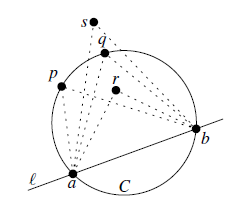Edge Flipping: 在一个三角化结果$\mathcal T$中，任取一条非边界边$e$，$e$两边的两个三角形共同构成一个以$e$为对角线的四边形。将$e$替换为该四边形的另一条对角线后，将会得到另一个三角化结果$\mathcal T’$，这一替换操作称为一次edge flipping（边翻转）。边翻转会将两个三角形变为另外两个三角形。设翻转前两个三角形的六个内角为$\alpha_1, \dots, \alpha_6$，翻转后为$\alpha_1’, \dots, \alpha_6’$，称$e$是一条非法边，当且仅当$\min_i\alpha_i < \min_i\alpha_i’$。

Theorem. 设$\mathcal T$包含非法边$e$，$\mathcal T’$是翻转$e$的结果，则$A(\mathcal T’) > A(\mathcal T)$。

while there exists an illegal edge e
flip e


### Delaunay Triangulation

Delaunay Graph: 给定平面点集$P$，$\mathrm{Vor}(P)$的对偶图称为$P$的Delaunay Graph，记作$\mathcal {DG}(P)$。

Theorem. Delaunary图总是可平面图。

General Position: 称一个点集是“in general position”的，当且仅当其中任意四个点都不在同一个圆上。这个概念用作点集的临时假设，可以简化算法叙述。